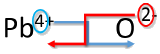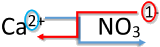# 5.5: Writing Formulas for Ionic Compounds

Learning Objectives

• Write the correct formula for an ionic compound.
• Recognize polyatomic ions in chemical formulas.

Ionic compounds do not exist as molecules. In the solid state, ionic compounds are in crystal lattice containing many ions each of the cation and anion. An ionic formula, like $$\ce{NaCl}$$, is an empirical formula. This formula merely indicates that sodium chloride is made of an equal number of sodium and chloride ions. Sodium sulfide, another ionic compound, has the formula $$\ce{Na_2S}$$. This formula indicates that this compound is made up of twice as many sodium ions as sulfide ions. This section will teach you how to find the correct ratio of ions, so that you can write a correct formula.

If you know the name of a binary ionic compound, you can write its chemical formula. Start by writing the metal ion with its charge, followed by the nonmetal ion with its charge. Because the overall compound must be electrically neutral, decide how many of each ion is needed in order for the positive and negative charges to cancel each other out.

Example $$\PageIndex{1}$$: Aluminum Nitride and Lithium Oxide

Write the formulas for aluminum nitride and lithium oxide.

Solution

Write the formula for aluminum nitride Write the formula for lithium oxide
1. Write the symbol and charge of the cation (metal) first and the anion (nonmetal) second. $$\ce{Al^{3+}} \: \: \: \: \: \ce{N^{3-}}$$ $$\ce{Li^+} \: \: \: \: \: \ce{O^{2-}}$$
2. Use a multiplier to make the total charge of the cations and anions equal to each other.

total charge of cations = total charge of anions

1(3+) = 1(3-)

+3 = -3

total charge of cations = total charge of anions

2(1+) = 1(2-)

+2 = -2

3. Use the multipliers as subscript for each ion. $$\ce{Al_1N_1}$$ $$\ce{Li_2O_1}$$
4. Write the final formula. Leave out all charges and all subscripts that are 1. $$\ce{AlN}$$ $$\ce{Li_2O}$$

An alternative way to writing a correct formula for an ionic compound is to use the crisscross method. In this method, the numerical value of each of the ion charges is crossed over to become the subscript of the other ion. Signs of the charges are dropped.

Example $$\PageIndex{2}$$: The Crisscross Method for Lead (IV) Oxide

Write the formula for lead (IV) oxide.

Solution

Crisscross Method Write the formula for lead (IV) oxide
1. Write the symbol and charge of the cation (metal) first and the anion (nonmetal) second. $$\ce{Pb^{4+}} \: \: \: \: \: \ce{O^{2-}}$$
2. Transpose only the number of the positive charge to become the subscript of the anion and the number only of the negative charge to become the subscript of the cation.3. Reduce to the lowest ratio. $$\ce{Pb_2O_4}$$
4. Write the final formula. Leave out all subscripts that are 1. $$\ce{PbO_2}$$

Exercise $$\PageIndex{2}$$

Write the chemical formula for an ionic compound composed of each pair of ions.

1. the calcium ion and the oxygen ion
2. the 2+ copper ion and the sulfur ion
3. the 1+ copper ion and the sulfur ion
CaO
CuS
Cu2S

Be aware that ionic compounds are empirical formulas and so must be written as the lowest ratio of the ions.

Example $$\PageIndex{3}$$: Sulfur Compound

Write the formula for sodium combined with sulfur.

Solution

Crisscross Method Write the formula for sodium combined with sulfur
1. Write the symbol and charge of the cation (metal) first and the anion (nonmetal) second.

$$\ce{Na^{+}} \: \: \: \: \: \ce{S^{2-}}$$

2. Transpose only the number of the positive charge to become the subscript of the anion and the number only of the negative charge to become the subscript of the cation.3. Reduce to the lowest ratio. This step is not necessary.
4. Write the final formula. Leave out all subscripts that are 1. $$\ce{Na_2S}$$

Exercise $$\PageIndex{3}$$

Write the formula for each ionic compound.

1. sodium bromide
2. lithium chloride
3. magnesium oxide
NaBr
LiCl
MgO

## Polyatomic Ions

Some ions consist of groups of atoms bonded together and have an overall electric charge. Because these ions contain more than one atom, they are called polyatomic ions. Polyatomic ions have characteristic formulas, names, and charges that should be memorized. For example, NO3 is the nitrate ion; it has one nitrogen atom and three oxygen atoms and an overall 1− charge. Table $$\PageIndex{1}$$ lists the most common polyatomic ions.

Table $$\PageIndex{1}$$: Some Polyatomic Ions
Name Formula
ammonium ion NH4+
acetate ion C2H3O2 (also written CH3CO2)
carbonate ion CO32−
chromate ion CrO42−
dichromate ion Cr2O72−
hydrogen carbonate ion (bicarbonate ion) HCO3
cyanide ion CN
hydroxide ion OH
nitrate ion NO3
nitrite ion NO2
permanganate ion MnO4
phosphate ion PO43−
hydrogen phosphate ion HPO42−
dihydrogen phosphate ion H2PO4
sulfate ion SO42−
hydrogen sulfate ion (bisulfate ion) HSO4
sulfite ion SO32−

The rule for constructing formulas for ionic compounds containing polyatomic ions is the same as for formulas containing monatomic (single-atom) ions: the positive and negative charges must balance. If more than one of a particular polyatomic ion is needed to balance the charge, the entire formula for the polyatomic ion must be enclosed in parentheses, and the numerical subscript is placed outside the parentheses. This is to show that the subscript applies to the entire polyatomic ion. An example is Ba(NO3)2.

## Writing Formulas for Ionic Compounds Containing Polyatomic Ions

Writing a formula for ionic compounds containing polyatomic ions also involves the same steps as for a binary ionic compound. Write the symbol and charge of the cation followed by the symbol and charge of the anion.

Example $$\PageIndex{4}$$: Calcium Nitrate

Write the formula for calcium nitrate.

Solution

Crisscross Method Write the formula for calcium nitrate
1. Write the symbol and charge of the cation (metal) first and the anion (nonmetal) second. $$\ce{Ca^{2+}} \: \: \: \: \: \ce{NO_3^-}$$
2. Transpose only the number of the positive charge to become the subscript of the anion and the number only of the negative charge to become the subscript of the cation.3. Reduce to the lowest ratio.

$$\ce{Ca_1(NO_3)_2}$$

4. Write the final formula. Leave out all subscripts that are 1. If there is only 1 of the polyatomic ion, leave off parentheses. $$\ce{Ca(NO_3)_2}$$

Example $$\PageIndex{5}$$

Write the chemical formula for an ionic compound composed of the potassium ion and the sulfate ion.

Solution

Potassium ions have a charge of 1+, while sulfate ions have a charge of 2−. We will need two potassium ions to balance the charge on the sulfate ion, so the proper chemical formula is K2SO4. $$K_2SO_4$$

Exercise $$\PageIndex{5}$$

Write the chemical formula for an ionic compound composed of each pair of ions.

1. the magnesium ion and the carbonate ion
2. the aluminum ion and the acetate ion
MgCO3
Al(CH3COO)3

## Recognizing Ionic Compounds

There are two ways to recognize ionic compounds. First, compounds between metal and nonmetal elements are usually ionic. For example, CaBr2 contains a metallic element (calcium, a group 2 [or 2A] metal) and a nonmetallic element (bromine, a group 17 [or 7A] nonmetal). Therefore, it is most likely an ionic compound. (In fact, it is ionic.) In contrast, the compound NO2 contains two elements that are both nonmetals (nitrogen, from group 15 [or 5A], and oxygen, from group 16 [or 6A]. It is not an ionic compound; it belongs to the category of covalent compounds discussed elsewhere. Also note that this combination of nitrogen and oxygen has no electric charge specified, so it is not the nitrite ion.

Second, if you recognize the formula of a polyatomic ion in a compound, the compound is ionic. For example, if you see the formula Ba(NO3)2, you may recognize the “NO3” part as the nitrate ion, NO3. (Remember that the convention for writing formulas for ionic compounds is not to include the ionic charge.) This is a clue that the other part of the formula, Ba, is actually the Ba2+ ion, with the 2+ charge balancing the overall 2− charge from the two nitrate ions. Thus, this compound is also ionic.

Example $$\PageIndex{6}$$

Identify each compound as ionic or not ionic.

1. Na2O
2. PCl3
3. NH4Cl
4. OF2

Solution

a. Sodium is a metal, and oxygen is a nonmetal. Therefore, Na2O is expected to be ionic. $$Na_2O$$, ionic
b. Both phosphorus and chlorine are nonmetals. Therefore, PCl3 is not ionic. $$PCl_3$$, not ionic
c. The NH4 in the formula represents the ammonium ion, NH4+, which indicates that this compound is ionic. $$NH_4Cl$$, ionic
d. Both oxygen and fluorine are nonmetals. Therefore, OF2 is not ionic. $$OF_2$$, ionic

Exercise $$\PageIndex{6}$$

Identify each compound as ionic or not ionic.

1. N2O
2. FeCl3
3. (NH4)3PO4
4. SOCl2
not ionic
ionic
ionic
not ionic

## Summary

Formulas for ionic compounds contain the symbols and number of each atom present in a compound in the lowest whole number ratio.